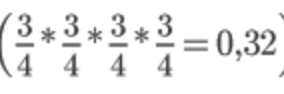## What is the probability of producing a child that will phenotypically

GeometryWhat is the probability of producing a child that will phenotypically2 years ago
What is the probability of producing a child that will phenotypically resemble either one of the two parents in the following four crosses? How many phenotypically different kinds of progeny could potentially result from each of the four crosses? Aa Bb Cc Dd * Aa Bb Cc Dd
If both parents have the genotype Aa Bb Cc Dd, their phenotype is determined by the dominant alleles for these four traits. If we observe on trait, we have 3 times bigger possibility for a dominant (AA, Aa, and Aa) than recessive phenotype (aa). Since we observe four different traits, the probability for an ofFSPring to look like their parents isThe number of possible phenotypes is 2 for each of the 4 traits (for example Aa and aa for the first trait), which makes it 16 (2 2 2 * 2).